#### You may also like### Pick's Theorem

Polygons drawn on square dotty paper have dots on their perimeter (p) and often internal (i) ones as well. Find a relationship between p, i and the area of the polygons.

# Pinned Squares

##### Age 14 to 16Challenge Level

Pin those squares down! A geoboard is a piece of wood with pins hammered onto it. The diagram shows a 5 by 5 board with 25 pins set out in a square array. Squares are made by stretching rubber bands round specific pins as shown. What is the total number of squares that can be made on a 5 by 5 board? How many squares can be made on an $N$ by $N$ board?

Ling Xiang Ning has illustrated his solution for $N = 2$, $3$ , $4$ and $5$ in a good clear diagram. There are 50 squares on a 5 by 5 board Can you find a general formula for an $N$ by $N$ board?

We count the squares on a 2 by 2 board, 3 by 3 board...

C by C board Total number of squares
2 by 2 1 ( 1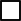)
3 by 3 6(4+ 1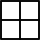+ 1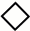)
4 by 4 20 ( 9+4+4+ 1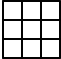+ 2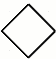)
5 by 5 50 ( 16+ 9+ 9+ 4+ 1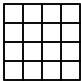+1+8+2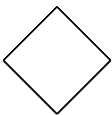)

Congratulations to Asher Walkover, age 17, of Woodmere, New York for finding the general formula for an $n$ by $n$ board by counting cases and summing series. Congratulations also to Sheila Luk and Joyce Fu for finding it by recognising, from the pattern of the first few terms and constructing a difference table, that the formula would be a polynomial, and using the results from the first few terms to find the coefficients.

Asher's method depends on counting the number of possible positions for the two 'leftmost' vertices of the squares formed. Here is Asher's solution:

Every oblique square made on the pinboard lies above and to the right of one of its edges which forms the hypotenuse of a right angled triangle with 2 legs, both less than $n$. Referring to the lengths of the legs, one leg may be 0, in which case the square will lie along the lines of the geoboard.

Consider the case where the horizontal leg of the triangle is $n - 1$, spanning $n$ nails. Clearly the vertical leg must be 0 and there is only one such triangle.

Now consider if the horizontal leg is $n - 2$, spanning $n - 1$ nails, then the vertical leg is either 0 or 1. If it is 0 there are 4 possible locations for the square, if it is 1 there is 1.

If the horizontal leg is $n - 3$ the vertical leg is 0, 1 or 2. If it is 0 there are 9, locations if 1 there are 4, if 2 there is 1.

The solution to this problem, as the pattern continues, will be: $$S = (1) + (1 + 4) + (1 + 4 + 9) + (1 + 4 + 9 + 16) + ... + (1 + 4 + 9 + (n - 2)^2 + (n - 1)^2).$$Note the pattern ends at $n - 1$ corresponding to the smallest square of side length 1 unit which has $(n - 1)^2$ possible positions.

Since the formula for $(1 + 4 + 9 + 16 + ... + n^2$) is $(n(n + 1)(2n + 1))/6$ or $(2n^3 + 3n^2 +n)/6$, all I had to do was use this to find the sum of the series $S$. Writing $m = n - 1$ this is:
\begin{eqnarray} \\ S &=& \sum_{k=1}^m k^3/3 + k^2/2 + k/6. \\ &=& (1/3)\sum_{k=1}^m k^3 + (1/2)\sum_{k=1}^m k^2 + (1/6)\sum_{k=1}^m k. \end{eqnarray}
Using the formulae
\begin{eqnarray} \\ 1 + 8 + 27 ... + m^3 &=& (m(m + 1)/2)^2 \\ 1 + 4 + 9 ... + m^2 &=& m(m + 1)(2m + 1)/6\\ 1 + 2 + 3 ... + m &=& m(m + 1)/2 \end{eqnarray}
I now have: $$S = [(m(m + 1))^2 + m(m + 1)(2m + 1) + m(m + 1)]/12$$which reduces rather nicely to $(m^4 + 4m^3 + 5m^2 + 2m)/12.$

Thus, if we have an $n$ by $n$ geoboard, and $m = n - 1$, the number of squares will be $$(m^4 + 4m^3 +5m^2 +2m)/12 = (n^4 - n^2)/12.$$ This can easily be verified for $n = 2, 3, 4$ and $5.$

Sheila and Joyce constructed this difference table for the numbers of squares formed and used it to find the general formula as follows: "

 1 6 20 50 105 196 5 14 30 55 91 9 16 25 36 7 9 11 2 2

Assuming the general formula is a quartic polynomial $an^4 +bn^2 +cn^3 + dn + e$ because the fourth differences appear to be constant, this leads to 5 simultaneous equations:
\begin{eqnarray} \\ a + b + c + d + e &=& 0 \\ 16a + 8b +4 c + 2d + e &=& 1 \\ 81a + 27b + 9c + 3d + e &=& 6 \\ 256a + 64b + 16c + 4d + e &=& 20 \\ 625a + 125b + 25c +5d + e &=& 50. \end{eqnarray}
Solving these equations gives the number of squares on an $n$ by $n$ board to be $(n^4 -n^2)/12$.